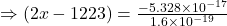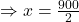A system of 1223 particles, each of which is either an electron or a proton, has a net charge of -5.328×10-17 C. How many protons are in thi

Question

A system of 1223 particles, each of which is either an electron or a proton, has a net charge of -5.328×10-17 C. How many protons are in this system (exactly)?

in progress 0
5 months 2021-09-02T15:18:04+00:00 1 Answers 0 views 0

Therefore the number of proton in the given system is 450.

Explanation:

Given that, a system has 1223 particles.

Let x number of proton be present in the system.

Then the number of electron is =(1223-x)

The charge of a proton is = 1.602×10⁻¹⁹ C

The charge of an electron = – 1.602×10⁻¹⁹ C

The charge of x protons is =( 1.6×10⁻¹⁹×x) C

The charge of (1223-x) electrons is = – 1.6×10⁻¹⁹ (1223-x) C

According to the problem,

(1.6×10⁻¹⁹×x) +{ – 1.6×10⁻¹⁹ (1223-x)}= -5.328×10⁻¹⁷

⇒1.6×10⁻¹⁹(x-1223+x)=-5.328×10⁻¹⁷⇒2x-1223= -333

⇒2x= -333+1223

⇒2x=900⇒x=450

Therefore the number of proton is 450.